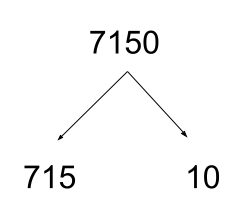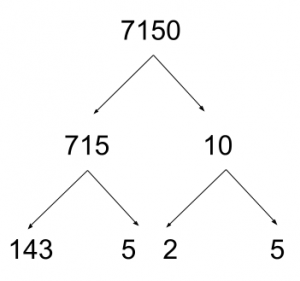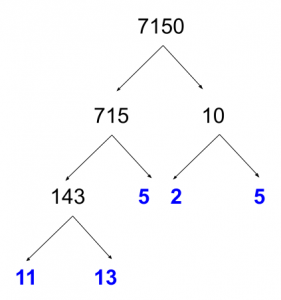By: Rich Zwelling (Apex GMAT Instructor)
Date: 1st April 2021

First, if you’d prefer to go straight to the explanation for the solution to the problem given in the last post, continue to the end of this post. But we’ll start with the following Official Guide GMAT problem as a way to talk about GMAT Prime Factorization. Give the problem a shot, if you can:

How many prime numbers between 1 and 100 are factors of 7,150?

A) One
B) Two
C) Three
D) Four
E) Five

One of the things you’ll notice is the linguistic setup of the problem, which is designed to confuse you immediately (a common theme on GMAT problems). They get you panicking right away with mention of a large range (between 1 and 100), and then they compound your frustration by giving you a rather large value (7,150).

Don’t let that convince you that you can’t do the problem. Because remember, the GMAT is not interested in large calculations, memorization involving large numbers, or weird arcana. Chances are, if you find yourself thinking about a complicated way to do a problem, you’re taking the wrong approach, and there’s a simpler way.

Try to pick out the most operative signal words, which let you know how to address the problem. We are dealing with prime numbers and also the topic of finding factors. The language of the problem may make you nervous, thinking that we must consider a slew of prime numbers up to 100. But the only primes we are really interested in are those that are actually factors of 7,150.

So let’s focus our attention there. And we can do so with a prime factor tree. Does this bring back memories?

Now, in the case of 7,150, we don’t have to break it down into prime numbers immediately. Split the number up into factors that are easy to recognize. In this case, the number ends in a zero, which means it is a multiple of 10, so we can start our tree like this:Notice that the advantage here is two-fold: It’s easier to divide by 10 and the two resulting numbers are both much more manageable.

Splitting up 10 into it’s prime factorization is straightforward enough (2 and 5). However, how do we approach 715? Well, it’s since it ends in a 5, we know it must be divisible by 5. At that point, you could divide 715 by 5 using long or short division…

…or you could get sneaky and use a NARRATIVE approach with nearby multiples:

750 is nearby, and since 75/5 = 15, that must mean that 750/5 = 150. Now, 750 is 35 greater than 715. And since 35/5 = 7, that means that 715 is seven multiples of 5 away from 750. So we can take the 150, subtract 7, and get 143

Mathematically, you can also see this as:

715/5 = (750-35)/5 = 750/5 – 35/5 = 150 – 7 = 143

So as stands, here’s our GMAT prime factorization:Now, there’s just the 143 to deal with, and this is where things get a bit interesting. There are divisibility rules that help make factoring easier, but an alternative you can always use is finding nearby multiples of the factor in question.

For example, is 143 divisible by 3? There is a rule for divisibility by 3, but you could also compare 143 against 150. 150 is a multiple of 3, and 143 is a distance of 7 away. 7 is not a multiple of 3, and therefore 143 is not a multiple of 3.

This rule applies for any factor, not just 3.

Now we can test the other prime numbers. (Don’t test 4 and 6, for example. We know 143 is not even, so it’s not divisible by 2. And if it’s not divisible by 2, it can’t be divisible by 4. Likewise, it’s not divisible by 3, so it can’t be divisible by 6, which is a multiple of 3.)

143 is not divisible by 5, since it doesn’t end in a 5 or 0. It’s not divisible by 7, since 140 is divisible by 7, and 143 is only 3 away.

What about 11? Here you have two options:

1. Think of 143 as 110+33, which is 11*10 + 11*3 → 11*(10+3) → 11*13
2. If you know your perfect squares well, you could think of 143 as 121+22

→ 11*11 + 11*2 → 11*(11+2) → 11*13

Either way, you should arrive at the same prime factorization:Notice that I’ve marked all prime numbers in blue. This result shouldn’t be a surprise, because notice that everything comes relatively clean: there are only a few prime numbers, they are relatively small, and there is just one slight complication in solving the problem (the factorization of 143).

So what is the answer? Be very careful that you don’t do all the hard work and falter at the last second. There are five ends to branches in the above diagram, which could lead you prematurely to pick answer choice E. But two of these branches have the same number (5). There are actually only four distinct primes (2, 5, 11, 13). The correct answer is D.

And again, notice that the range given in the question stem (1 to 100) is really a linguistic distraction to throw you off track. We don’t even go beyond 13.

Next time, we’ll talk about the fascinating topic of twin primes and how they connect to divisibility.